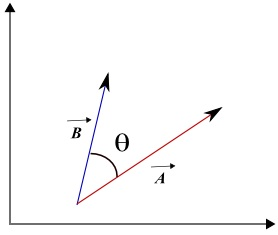# Scalar Product of Vectors

 Scalar Product of Vectors The scalar product (also called the dot product and inner product) of vectors A and B is written and defined as followsFig1. - Angle between vectors and scalar product. A�B = | A | | B | cos (θ) where | A | is the magnitude of vector A , | B | is the magnitude of vector B and θ is the angle made by the two vectors. The result of a scalar product of two vectors is a scalar quantity. For vectors given by their components: A = (Ax , Ay, Az) and B = (Bx , By, Bz), the scalar product is given by A�B = AxBx + AyBy + AzBz Note that if θ = 90�, then cos(θ) = 0 and therefore we can state that: Two vectors, with magnitudes not equal to zero, are perpendicular if and only if their scalar product is equal to zero. The scalar product may also be used to find the cosine and therefore the angle between two vectors cos (θ) = A�B / | A | | B | Properties of the Scalar Product 1) A�B = B�A 2) A� (B + C) = A�B + A�C Applications the Scalar Product Question 1 Find the real number b so that vectors A and B given below are perpendicular A = (-2 , -b) , B = (-8 , b). Solution to Question 1 The condition for two vectors A = (Ax , Ay) and B = (Bx , By) to be perpendicular is that their scalar product is equal to zero: AxBx + AyBy = 0. Substitute the components and simplify (-2)(-8) + (-b)(b) = 0 16 - b 2 = 0 b 2 = 16 Solve for b b = 4 and b = -4 Two possible values of b; b = 4 and b = - 4 make the above vectors perpendicular. Question 2 Find the angle made by the vectors A and B given below A = (2 , 1 , 3) , B = (3 , -2 , 1). Solution to Question 2 We first use the components to find the scalar product of the two vectors. A� B = (2)(3)+(1)(-2)+(3)(1) = 7 We next express the scalar product using the magnitudes and angle θ made by the two vectors. A� B = |A| |B| cos(θ) = 7 Which gives cos(θ) = 7 / (|A| |B|) |A| = √(22 + 12 + 32 ) = √14 |B| = √(32 + (-2)2 + 12 ) = √14 cos(θ) = 7 / (√14√14) = 7/14 = 1/2 θ = arccos(1/2) = 60� Question 3 Given vector U = (3 , -7), find the equation of the line through point B(2 , 1) and perpendicular to vector U. Solution to Question 3 A point M(x , y) is on the line through point B(2 , 1) and perpendicular to vector U = (3 , -7) if and only if the vectors BM and U are perpendicular. Let us first find the components of vectors BM. BM = (x - 2 , y - 1) Vectors BM = (x - 2 , y - 1) and U = (3 , -7) are perpendiclur if and only if their scalar product is equal to zero. Hence (x - 2) (3) + (y - 1)(-7) = 0 Expand and simplify to obtain the equation of the line 3 x - 7 y = - 1 Question 4 Given points A(1 , 2) and B(-2 , -2), find the equation of the tangent at point B to the circle with diameter AB.Fig1. - Tangent to circle. Solution to Question 4 A tangent to a circle at point B is perpendicular to segment BC where C is the center of the circle (see figure on the right). Any point M(x , y) on the tangent is such that the scalar product BM�BC is equal to zero. Point C is the center of the circle and therefore the midpoint of A and B . Its coordinates are given by C( (1+(-2)) / 2 , (2 + (-2)) / 2) = C(-1 / 2 , 0) Vectors BM and BC are defined by points B and M and B and C and their components are given by BM = (x - (-2)) , y - (-2)) = (x + 2 , y + 2) BC = (-1 / 2 - (-2)) , 0 - (-2)) = (3 / 2 , 2) We now use the fact that the scalar product is equal to zero. BM�BC = (x + 2)(3/2) + (y + 2)(2) = 0 Expand and simplify to find the equation of the tangent. 3 x / 2 + 2 y = - 7 Question 5 Find the angle between the lines given by the equations: y = 2 x + 4 and y = x + 3.Fig1. -Angle between two lines . Solution to Question 5 Let L1 be the line with equation y = 2 x + 4 and line L2 the line with equation L2. First find the point of intersection by solving the system of equations: y = 2 x + 4 and y = x + 3. The point of intersection is at (-1 , 2) We now find the y-intercepts of the two lines For line L1 the y-intercept is (0 , 4) and for L2 the y-intercept is(0 , 3) We now find two vectors V1 and V2 parallel to L1 and L2 respectively. (see figure on the right) V1 = (0 - (-1) , 4 - 2) = (1 , 2) and V2 = (0 - (-1) , 3 - 2) = (1 , 1) We now calculate the angle θ θ = arccos [ (V1�V2) / (|V1| |V2| ) ] = arccos [ (1 , 2) � (1 , 1) / (√5 √2)] = 18.43�